# Vertex AI：分布式超参数调节

## 学习内容

• 在自定义容器中使用分布式训练来训练模型
• 启动训练代码的多次试验以自动进行超参数调节

## 2. Vertex AI 简介

Vertex AI 包含许多不同的产品，可支持端到端机器学习工作流。本实验将重点介绍 TrainingWorkbench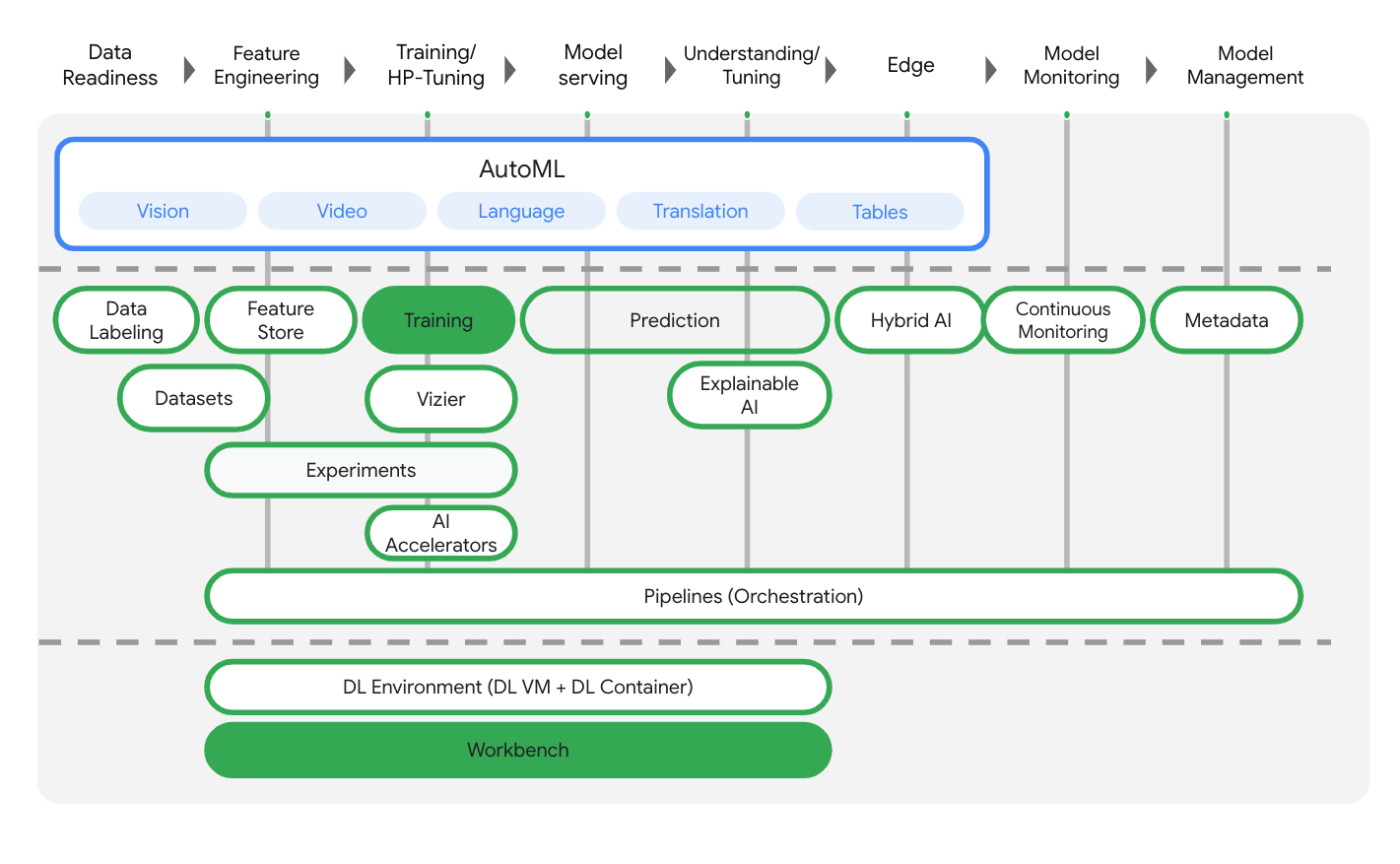## 3. 用例概览

#### 超参数调节

1. 在主训练模块中，为您要调节的每个超参数定义一个命令行参数。
2. 使用这些参数中传递的值，在应用的代码中设置相应的超参数。

## 第 3 步：启用 Vertex AI API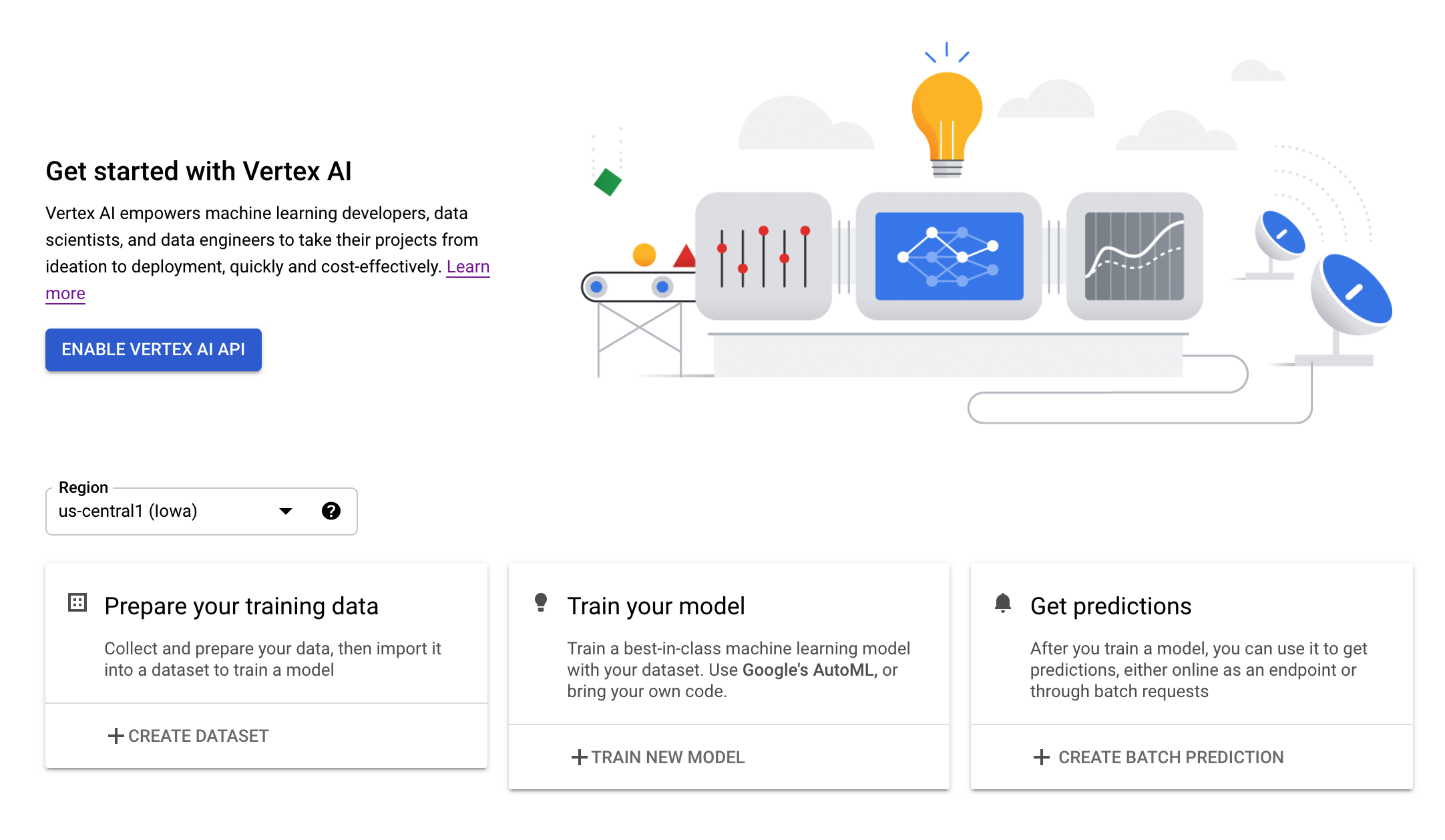## 第 4 步：创建 Vertex AI Workbench 实例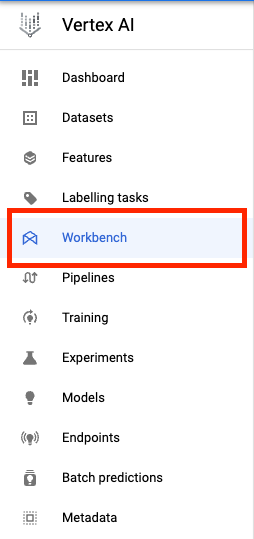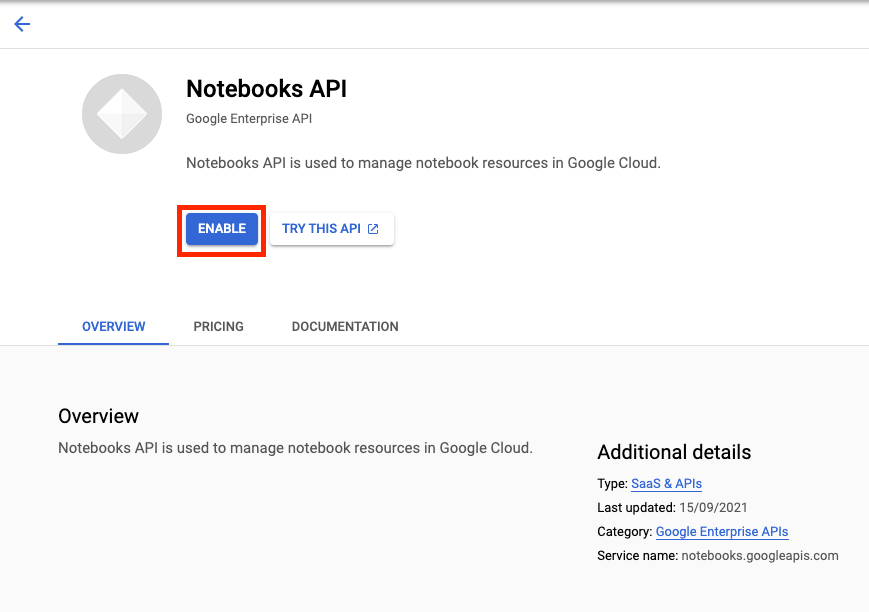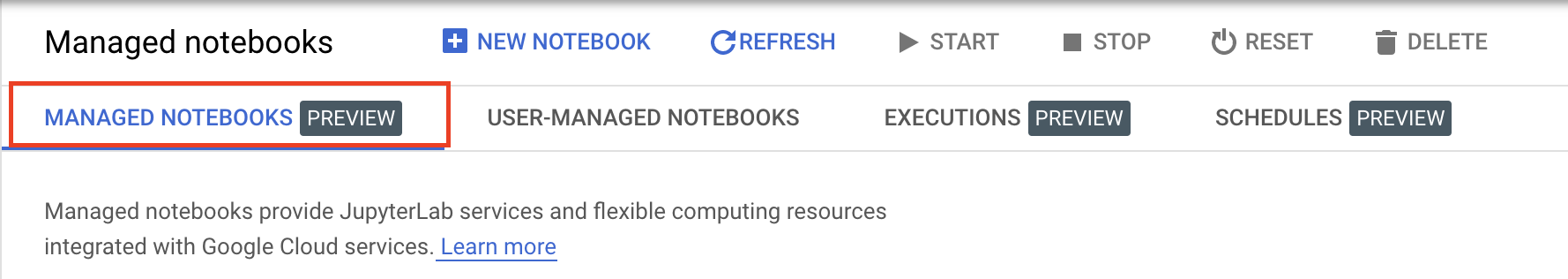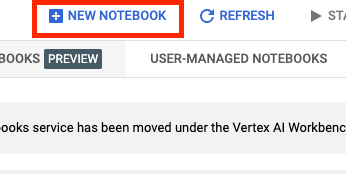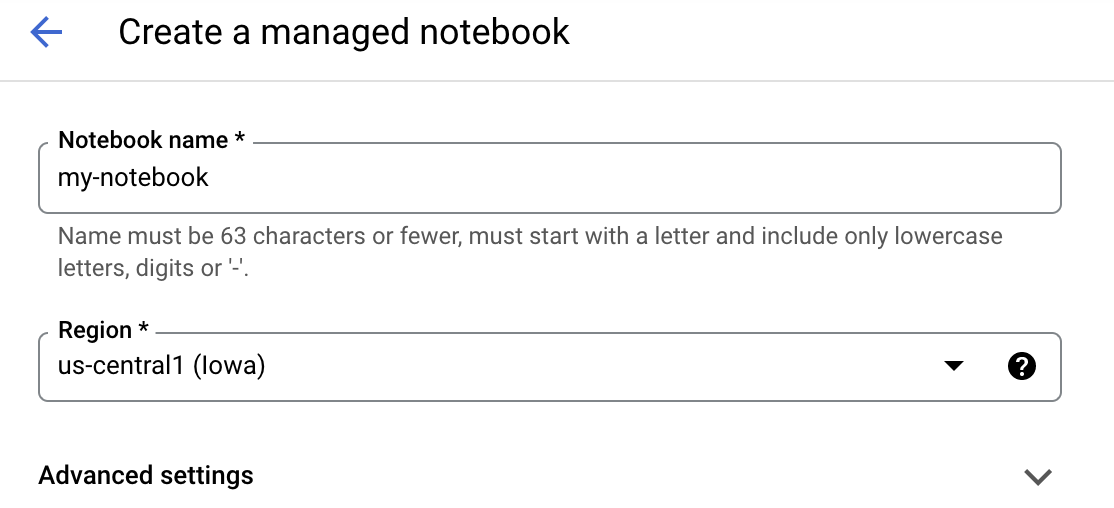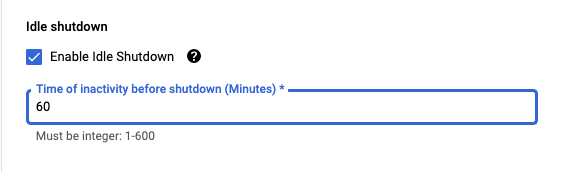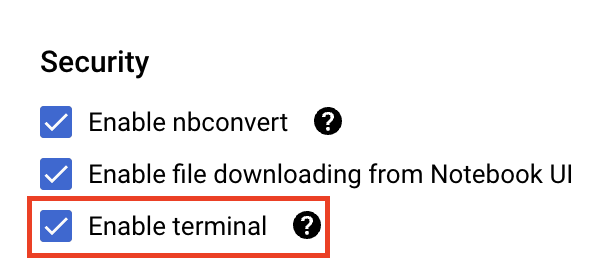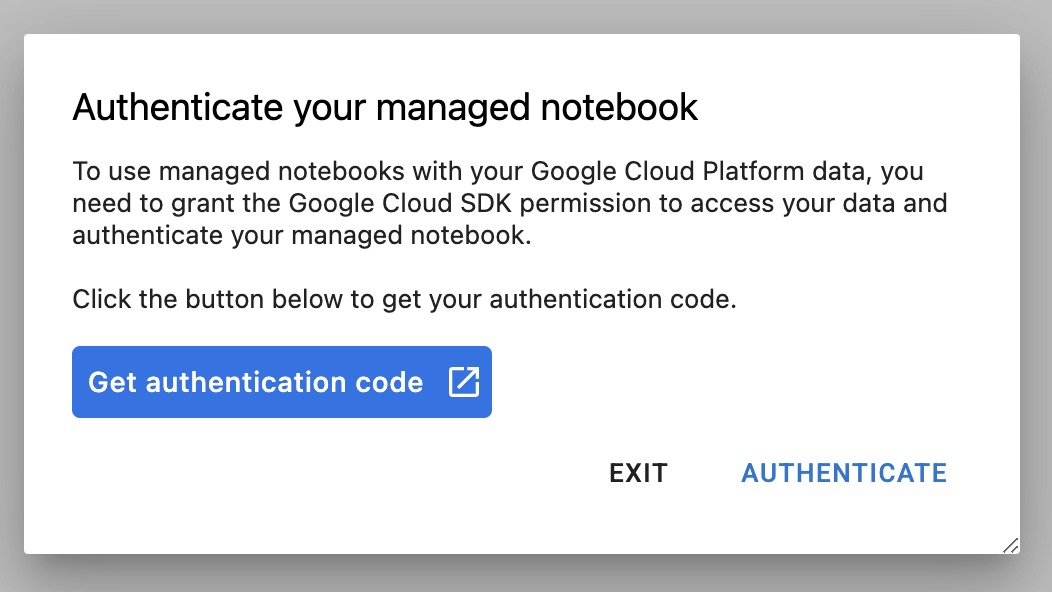## 5. 编写训练代码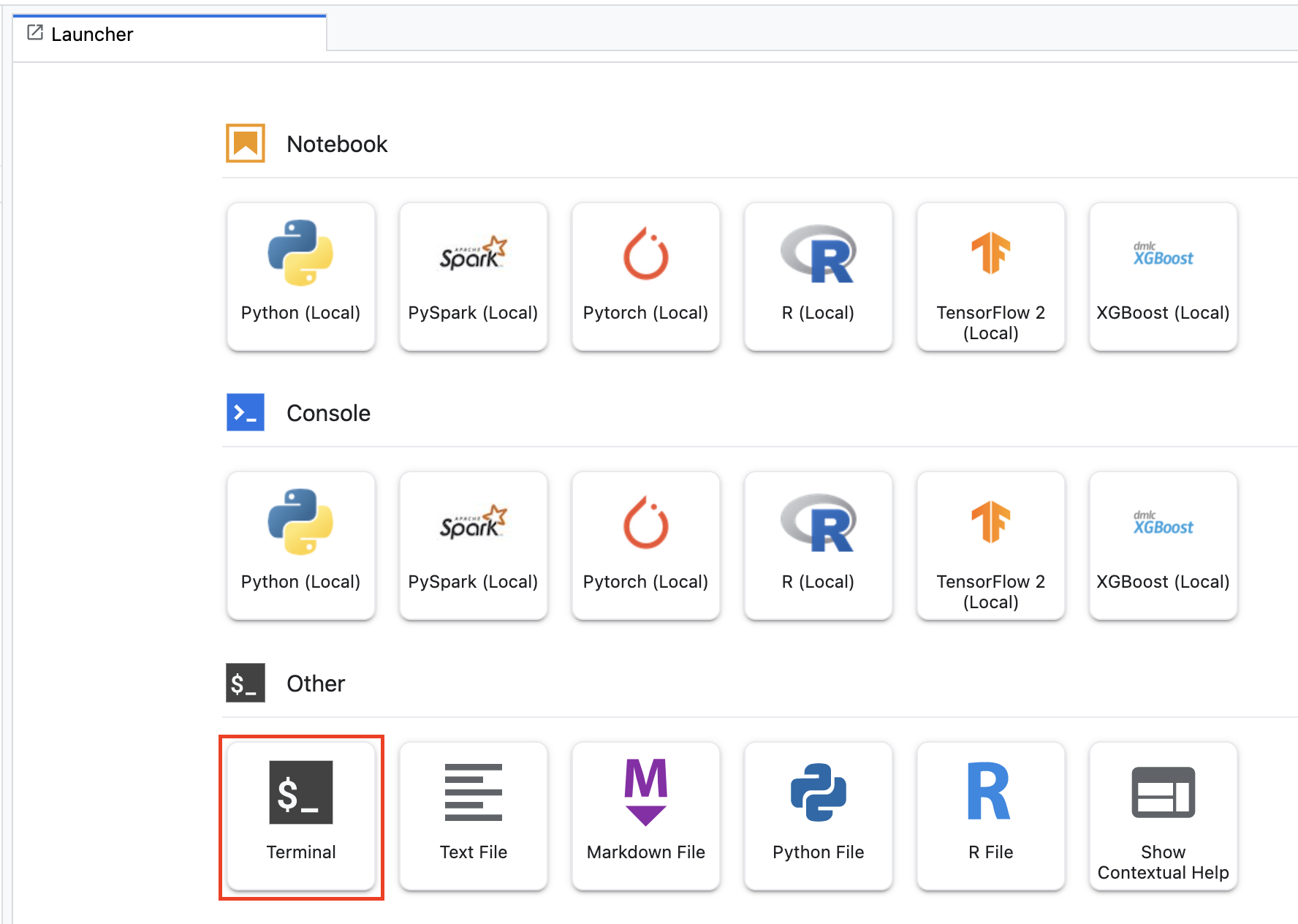``````mkdir vertex-codelab
cd vertex-codelab
``````

``````mkdir trainer
``````

``````+ trainer/
``````

``````import tensorflow as tf
import tensorflow_datasets as tfds
import argparse
import hypertune
import os

NUM_EPOCHS = 10
BATCH_SIZE = 64

def get_args():
'''Parses args. Must include all hyperparameters you want to tune.'''

parser = argparse.ArgumentParser()
'--learning_rate',
required=True,
type=float,
help='learning rate')
'--momentum',
required=True,
type=float,
help='SGD momentum value')
'--num_units',
required=True,
type=int,
help='number of units in last hidden layer')
args = parser.parse_args()
return args

def preprocess_data(image, label):
'''Resizes and scales images.'''

image = tf.image.resize(image, (150,150))
return tf.cast(image, tf.float32) / 255., label

def create_dataset(batch_size):
'''Loads Horses Or Humans dataset and preprocesses data.'''

data, info = tfds.load(name='horses_or_humans', as_supervised=True, with_info=True)

# Create train dataset
train_data = data['train'].map(preprocess_data)
train_data  = train_data.shuffle(1000)
train_data  = train_data.batch(batch_size)

# Create validation dataset
validation_data = data['test'].map(preprocess_data)
validation_data  = validation_data.batch(batch_size)

return train_data, validation_data

def create_model(num_units, learning_rate, momentum):
'''Defines and compiles model.'''

inputs = tf.keras.Input(shape=(150, 150, 3))
x = tf.keras.layers.Conv2D(16, (3, 3), activation='relu')(inputs)
x = tf.keras.layers.MaxPooling2D((2, 2))(x)
x = tf.keras.layers.Conv2D(32, (3, 3), activation='relu')(x)
x = tf.keras.layers.MaxPooling2D((2, 2))(x)
x = tf.keras.layers.Conv2D(64, (3, 3), activation='relu')(x)
x = tf.keras.layers.MaxPooling2D((2, 2))(x)
x = tf.keras.layers.Flatten()(x)
x = tf.keras.layers.Dense(num_units, activation='relu')(x)
outputs = tf.keras.layers.Dense(1, activation='sigmoid')(x)
model = tf.keras.Model(inputs, outputs)
model.compile(
loss='binary_crossentropy',
optimizer=tf.keras.optimizers.SGD(learning_rate=learning_rate, momentum=momentum),
metrics=['accuracy'])
return model

def main():
args = get_args()

# Create distribution strategy
strategy = tf.distribute.MirroredStrategy()

# Get data
GLOBAL_BATCH_SIZE = BATCH_SIZE * strategy.num_replicas_in_sync
train_data, validation_data = create_dataset(GLOBAL_BATCH_SIZE)

# Wrap variable creation within strategy scope
with strategy.scope():
model = create_model(args.num_units, args.learning_rate, args.momentum)

# Train model
history = model.fit(train_data, epochs=NUM_EPOCHS, validation_data=validation_data)

# Define metric
hp_metric = history.history['val_accuracy'][-1]

hpt = hypertune.HyperTune()
hpt.report_hyperparameter_tuning_metric(
hyperparameter_metric_tag='accuracy',
metric_value=hp_metric,
global_step=NUM_EPOCHS)

if __name__ == "__main__":
main()
``````

### 分布式训练

1. `main()` 函数中，相关代码创建了 `MirroredStrategy` 对象。接下来，您将模型变量的创建过程封装在策略的范围内。此步骤指示 TensorFlow 应在 GPU 中镜像哪些变量。
2. 批次大小按 `num_replicas_in_sync` 扩容。在 TensorFlow 中使用同步数据并行策略时，扩缩批次大小是最佳实践。您可以点击此处了解详情

### 超参数调节

1. 该脚本会导入 `hypertune` 库。之后，在构建容器映像时，我们需要确保安装此库。
2. `get_args()` 函数为您要调节的每个超参数定义命令行参数。在此示例中，将要调节的超参数是学习速率、优化器中的动量值以及模型最后一个隐藏层中的单元数，但您可以随意尝试其他超参数。然后，在这些参数中传递的值将用于在代码中设置相应的超参数（例如，设置 `learning_rate = args.learning_rate`）。
3. `main()` 函数的末尾，`hypertune` 库用于定义您要优化的指标。在 TensorFlow 中，Keras `model.fit` 方法会返回 `History` 对象。`History.history` 特性是连续周期的训练损失值和指标值的记录。如果您将验证数据传递给 `model.fit`，则 `History.history` 特性也将包含验证损失和指标值。例如，如果您使用验证数据将模型训练三个周期并提供 `accuracy` 作为指标，则 `History.history` 特性将类似于以下字典。
``````{
"accuracy": [
0.7795261740684509,
0.9471358060836792,
0.9870933294296265
],
"loss": [
0.6340447664260864,
0.16712145507335663,
0.04546636343002319
],
"val_accuracy": [
0.3795261740684509,
0.4471358060836792,
0.4870933294296265
],
"val_loss": [
2.044623374938965,
4.100203514099121,
3.0728273391723633
]
``````

## 第 1 步：编写 Dockerfile

``````touch Dockerfile
``````

``````+ Dockerfile
+ trainer/
``````

``````FROM gcr.io/deeplearning-platform-release/tf2-gpu.2-7

WORKDIR /

# Installs hypertune library
RUN pip install cloudml-hypertune

# Copies the trainer code to the docker image.
COPY trainer /trainer

# Sets up the entry point to invoke the trainer.
``````

## 第 2 步：构建容器

``````PROJECT_ID='your-cloud-project'
``````

``````IMAGE_URI="gcr.io/\$PROJECT_ID/horse-human-codelab:latest"
``````

``````gcloud auth configure-docker
``````

``````docker build ./ -t \$IMAGE_URI
``````

``````docker push \$IMAGE_URI
``````

## 第 3 步：创建 Cloud Storage 存储桶

``````BUCKET_NAME="gs://\${PROJECT_ID}-hptune-bucket"
gsutil mb -l us-central1 \$BUCKET_NAME
``````

## 第 1 步：使用超参数调节创建自定义训练作业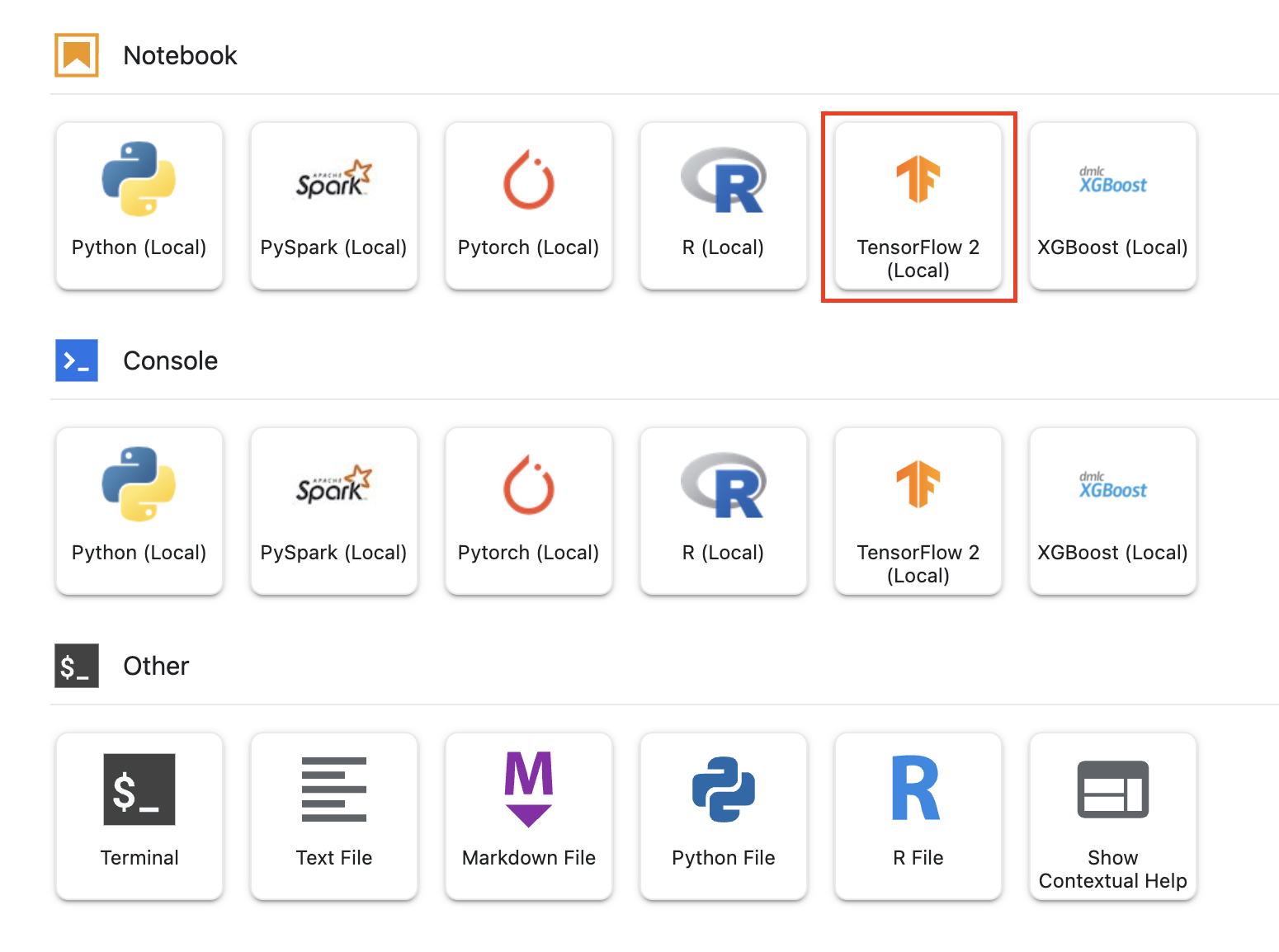``````from google.cloud import aiplatform
from google.cloud.aiplatform import hyperparameter_tuning as hpt
``````

``````# The spec of the worker pools including machine type and Docker image
# Be sure to replace PROJECT_ID in the "image_uri" with your project.

worker_pool_specs = [{
"machine_spec": {
"machine_type": "n1-standard-4",
"accelerator_type": "NVIDIA_TESLA_V100",
"accelerator_count": 2
},
"replica_count": 1,
"container_spec": {
"image_uri": "gcr.io/{PROJECT_ID}/horse-human-codelab:latest"
}
}]

``````

``````# Dictionary representing parameters to optimize.
# The dictionary key is the parameter_id, which is passed into your training
# job as a command line argument,
# And the dictionary value is the parameter specification of the metric.
parameter_spec = {
"learning_rate": hpt.DoubleParameterSpec(min=0.001, max=1, scale="log"),
"momentum": hpt.DoubleParameterSpec(min=0, max=1, scale="linear"),
"num_units": hpt.DiscreteParameterSpec(values=[64, 128, 512], scale=None)
}
``````

``````# Dicionary representing metrics to optimize.
# The dictionary key is the metric_id, which is reported by your training job,
# And the dictionary value is the optimization goal of the metric.
metric_spec={'accuracy':'maximize'}
``````

``````# Replace YOUR_BUCKET
my_custom_job = aiplatform.CustomJob(display_name='horses-humans',
worker_pool_specs=worker_pool_specs,
staging_bucket='gs://{YOUR_BUCKET}')
``````

``````hp_job = aiplatform.HyperparameterTuningJob(
display_name='horses-humans',
custom_job=my_custom_job,
metric_spec=metric_spec,
parameter_spec=parameter_spec,
max_trial_count=6,
parallel_trial_count=2,
search_algorithm=None)

hp_job.run()
``````

• max_trial_count：您需要对服务将运行的试验次数设置上限。更多的试验通常会带来更好的结果，但会有一个收益递减点，在该点之后，额外的试验对您尝试优化的指标几乎没有什么效果。最佳实践是从少量试验开始，并在扩容之前了解您所选超参数的影响力。
• parallel_trial_count：如果您使用并行试验，则服务会预配多个训练处理集群。增加并行试验的次数会减少超参数调节作业运行所需的时间，但可能降低作业的整体效率。这是因为默认调节策略使用先前试验的结果来告知后续试验中的赋值情况。
• search_algorithm：您可以将搜索算法设置为网格、随机或默认（无）。默认选项会应用贝叶斯优化来搜索可能的超参数值空间，并且是推荐的算法。如需详细了解此算法，请点击此处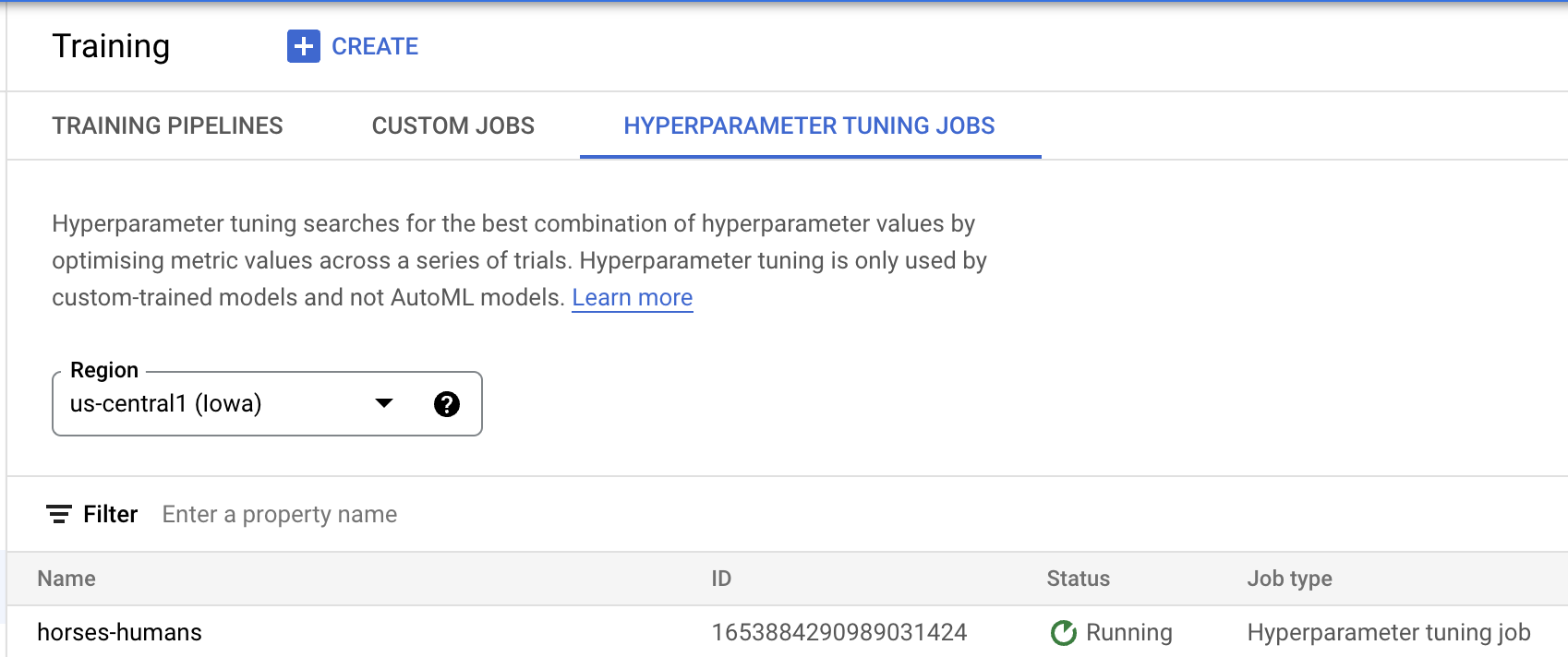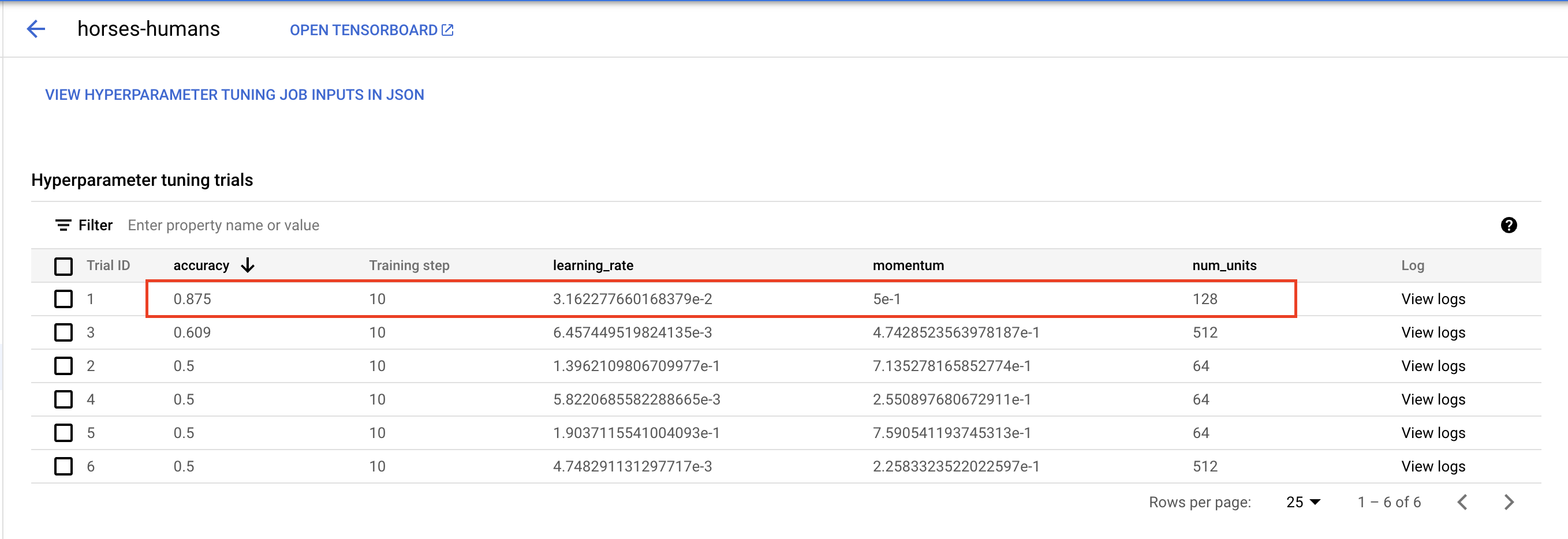🎉 恭喜！🎉

• 使用分布式训练运行超参数调节作业

## 8. 清理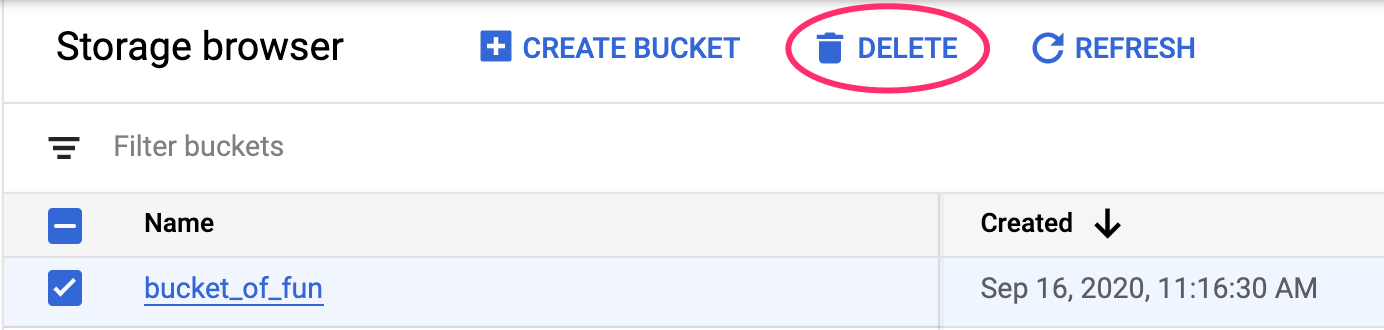[{ "type": "thumb-down", "id": "missingTheInformationINeed", "label":"没有我需要的信息" },{ "type": "thumb-down", "id": "tooComplicatedTooManySteps", "label":"太复杂/步骤太多" },{ "type": "thumb-down", "id": "outOfDate", "label":"内容需要更新" },{ "type": "thumb-down", "id": "translationIssue", "label":"翻译问题" },{ "type": "thumb-down", "id": "samplesCodeIssue", "label":"示例/代码问题" },{ "type": "thumb-down", "id": "otherDown", "label":"其他" }]
[{ "type": "thumb-up", "id": "easyToUnderstand", "label":"易于理解" },{ "type": "thumb-up", "id": "solvedMyProblem", "label":"解决了我的问题" },{ "type": "thumb-up", "id": "otherUp", "label":"其他" }]